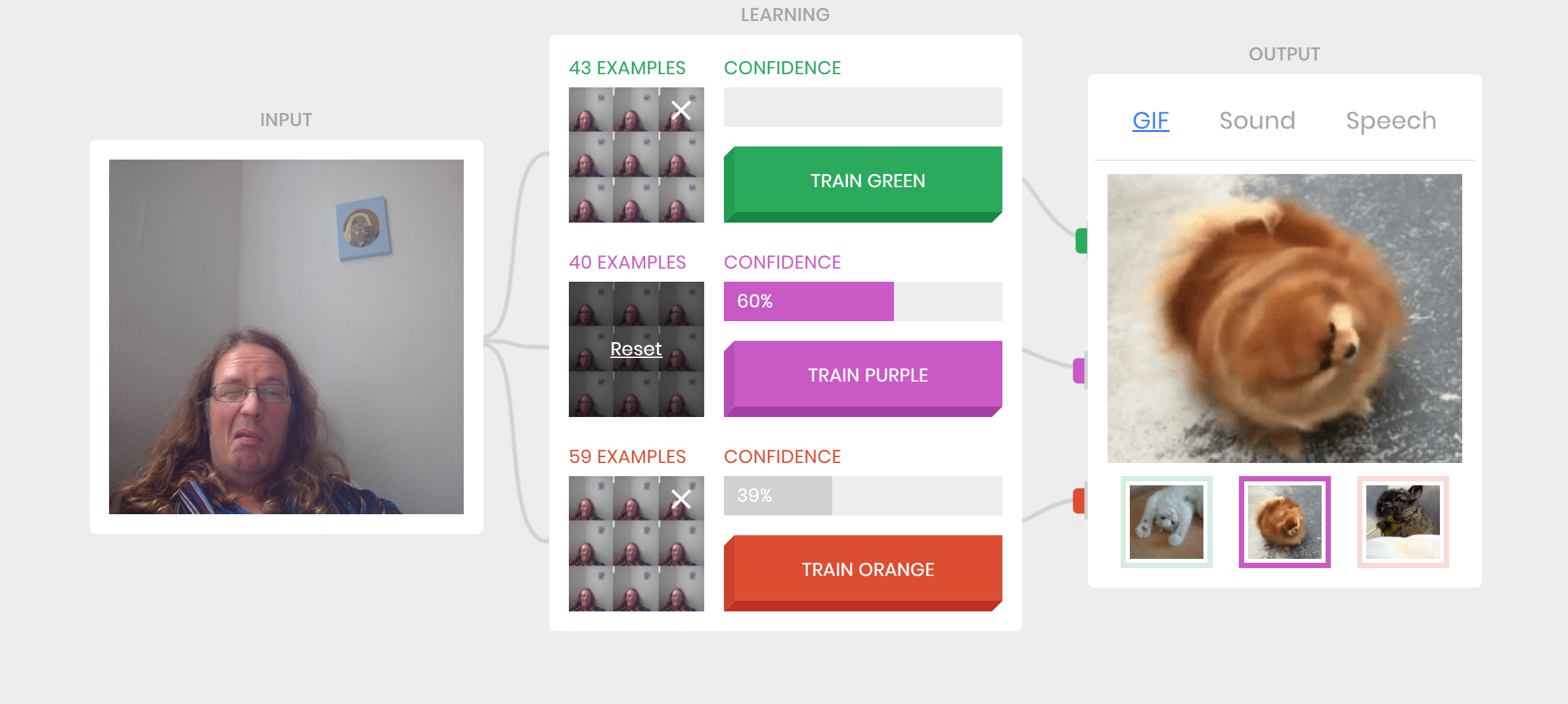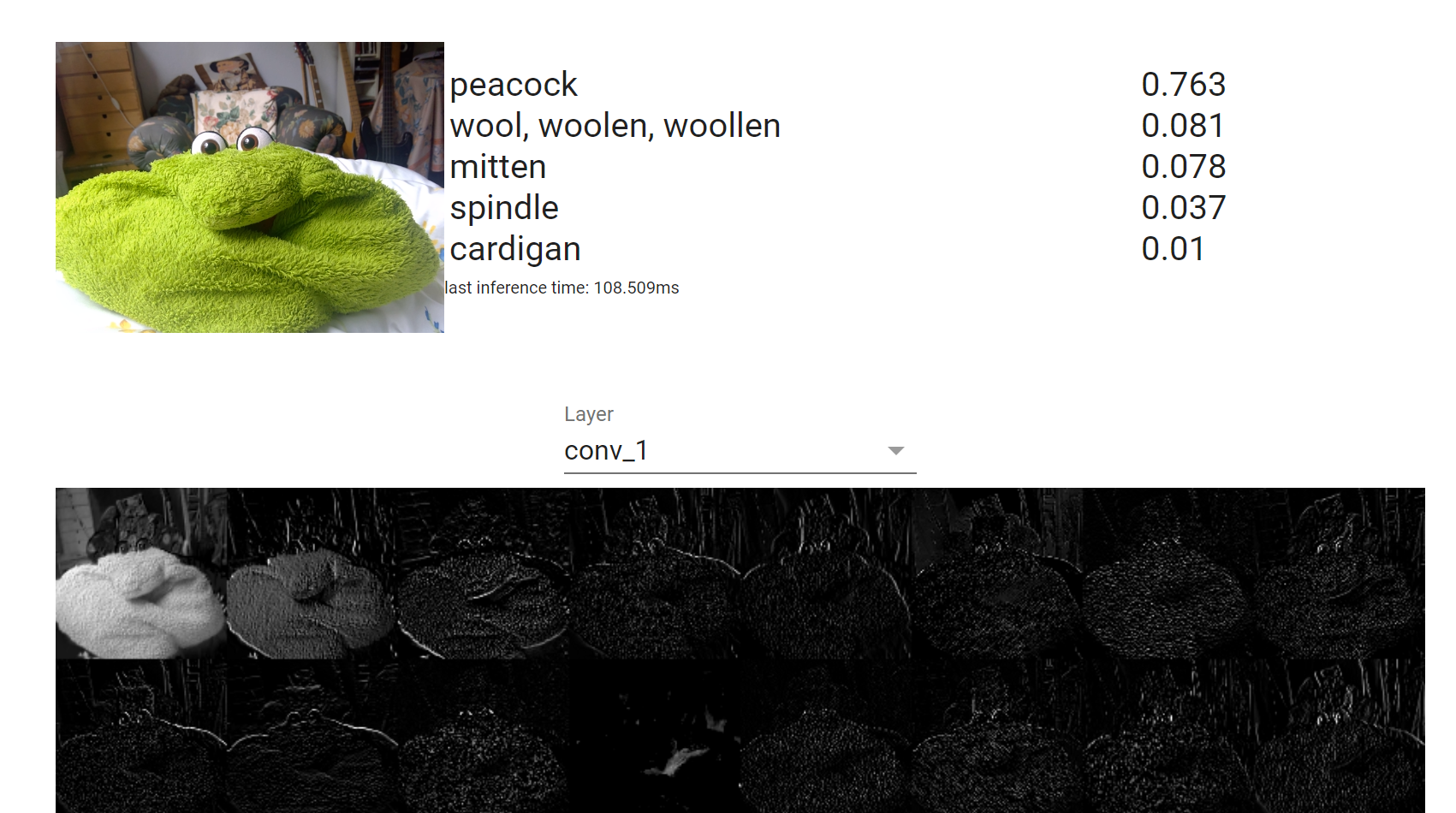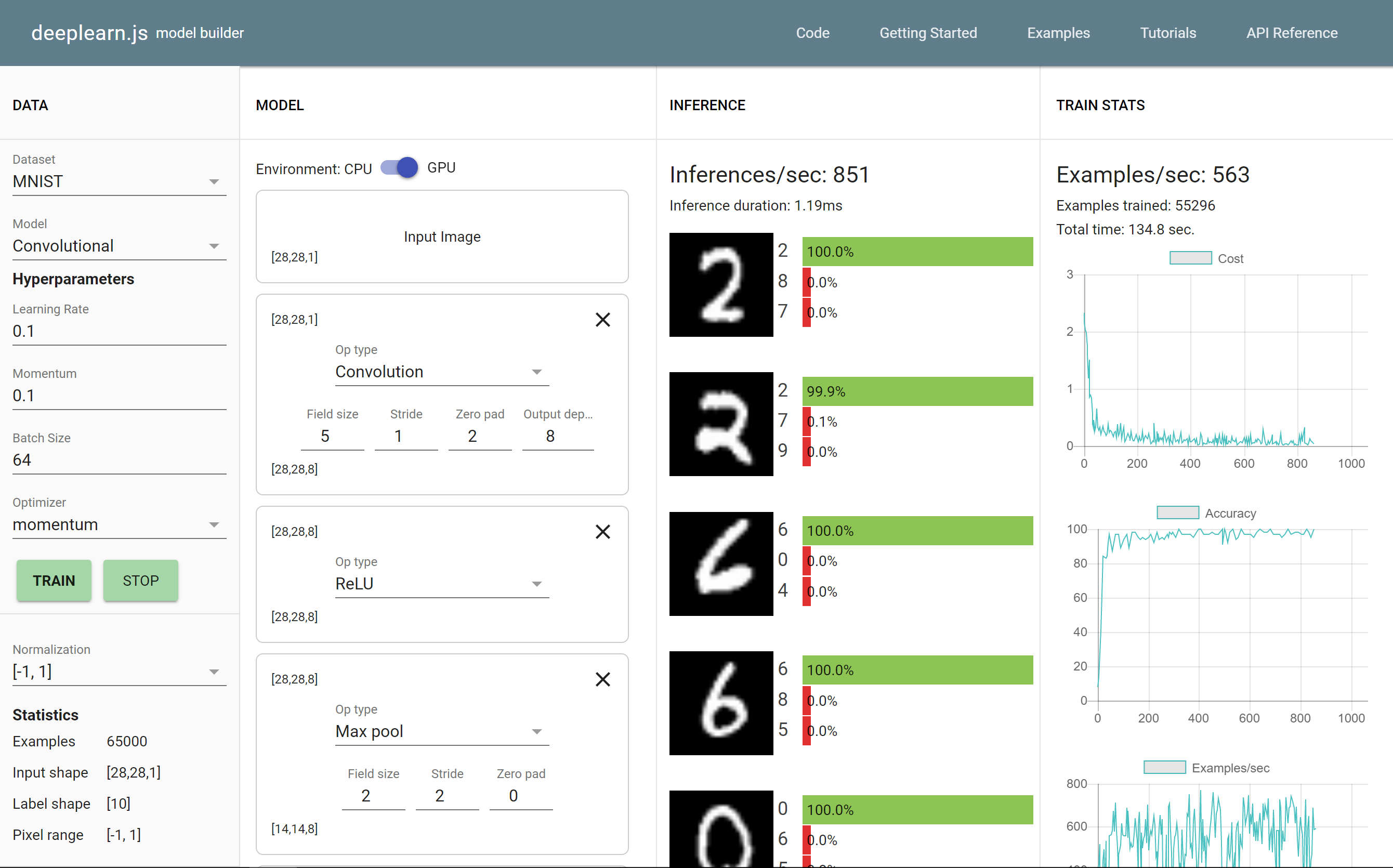## Machine Learning in the Browser

### Using deeplearn.js

#### Oliver Zeigermann / @DJCordhose

http://bit.ly/ml-hhjs

### Supervised Machine Learning

Surprisingly, despite AI’s breadth of impact, the types of it being deployed are still extremely limited. Almost all of AI’s recent progress is through one type, in which some input data (A) is used to quickly generate some simple response (B)

Andrew Ng: https://hbr.org/2016/11/what-artificial-intelligence-can-and-cant-do-right-now

### How does (Supervised) Machine learning work?

Built using deeplearn.js (more on that later in this talk), source code availablehttps://teachablemachine.withgoogle.com/
Part of Google AI Experiments

### deeplearn.js

Full TypeScript ML library using browser GPUhttps://pair-code.github.io/deeplearnjs

Includes full training mimicking TensorFlow and NumPy API https://research.googleblog.com/2017/08/harness-power-of-machine-learning-in.html

### Simple Example: Scalar times Array

``````
const math = new NDArrayMathGPU();
// const math = new NDArrayMathCPU();
``````
``````
const array = Array1D.new([1, 2, 3]);
const scalar = Scalar.new(2);
``````
``````
const result = math.scalarTimesArray(scalar, array);
console.log(await result.data());
// => Float32Array([2, 4, 6])
``````

### Our small project

Fit a polynomial curve through some data pointshttps://djcordhose.github.io/ai/js/deeplearn-sandbox

### First Step: Set up computational graph

`y = a * x2 + b * x + c`

``````
const graph = new Graph();
``````
``````
const x = graph.placeholder('x', []);
``````
``````
const a = graph.variable('a', Scalar.new(Math.random()));
const b = graph.variable('b', Scalar.new(Math.random()));
const c = graph.variable('c', Scalar.new(Math.random()));
``````
``````
const order2 = graph.multiply(a, graph.square(x));
const order1 = graph.multiply(b, x);

const y = graph.add(graph.add(order2, order1), c);
``````

### Second Step: Push it over to the GPU for processing

wildly asynchronous, but `async/await` help

``````
const math = new NDArrayMathGPU(); // could also be CPU
const session = new Session(graph, math);
``````
``````
const sampleX = 4;
math.scope(async (keep, track) => {
const result =
session.eval(y, [{ tensor: x,
data: track(Scalar.new(sampleX))
}]);
console.log(await result.get())
});
``````

GPU resource management is partially on us (`keep, track`)

### Step Three: Learning by minimizing Error

Long running, complex computations on GPU

``````
const yLabel = graph.placeholder('y label', []);
const cost = graph.meanSquaredCost(y, yLabel);
``````
``````
const LEARNING_RATE = .01;
const optimizer = new SGDOptimizer(LEARNING_RATE);
``````
``````
// repeat a number of times (epochs)
session.train(
cost,
// matching x/y pairs
[{ tensor: x, data: xProvider },
{ tensor: yLabel, data: yProvider }],
optimizer, CostReduction.MEAN);
``````

### Objectives Met

• Deploy (as Web App over GitHub): Zero Install, every GPU
• Educate: make it accessible to everyone
• Visualize: show how it works
• Develop: let's you develop in JavaScript

### deeplearn.js: Model Builder

Build a neural network in your browserhttps://deeplearnjs.org/demos/model-builder/

#### More Machine Learning with JavaScript

• Facets : data overview visualizations
• Keras.js : Running Keras Models in the Browser using GPU
• TensorFire : GPU based inference (no training) in the browser, runs Keras and TensorFlow models
• ConvNetJS: Visual NN exploration for learning (t-SNE cluster exploration from same auhtor)
• Brain.js : simple and straing forward NN implementation
• synaptic.js: similar to Brain.js, a bit more active
• ml.js: generic low level libs for machine learning

#### deeplearn.js has the potential to become the JavaScript library for Machine Learning

• can make use of any GPU (not only CUDA)
• has highest reach due to zero installation
• can be easily integrated into existing Web Apps
• can not load trained Keras or Tensorflow models
• coding style highly asynchronous
• GPU processing needs chunks of uninterrupted processing
• currently at 0.3.3, not quite ready for prime time, yet

Machine Learning in the Browser, hh.js 2017
Oliver Zeigermann / @DJCordhose
http://bit.ly/ml-hhjs

### More People being enthusiastic about Deeplearn.js

https://www.kickstarter.com/projects/rocksetta/joe-javascripts-machine-learning-tutorials